[ITOC-ch2] CONTEXT-FREE LANGUAGES

In this chapter we present context-free grammars to describe certain features that have a recursive structure.

help us organize and understand relationships of terms.

Application: compilers and interpreters

• Most compilers and interpreters contain a parser that extracts the meaning of a program before generating the compiled code or performing the interpreted execution.

2.1 CONTEXT-FREE GRAMMAR

Context Free Grammar

• consists of a collection of substitution rules

Variable

• symbols on the left hand side

Terminal

• other symbols
• can’t appear in the left hand side in CFG ( Ex: aB –> XX )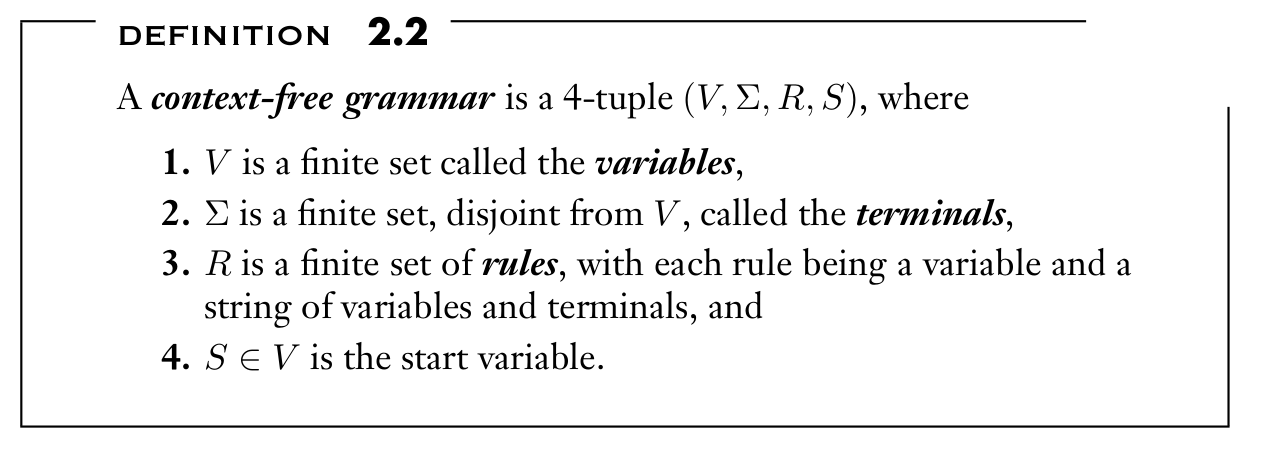Derivation

• The sequence of substitutions to obtain a string
• u derives v, written $u ⇒^{ * } v$, if u = v or if a sequence $u 1 , u 2 , . . . , u k$ exists for k ≥ 0 and

$u ⇒ u 1 ⇒ u 2 ⇒ . . . ⇒ u k ⇒ v.$

Context Free Language

• strings generated by CFG
• $\{ w ∈ Σ^{ ∗ } | S ⇒^{ * } w \}.$

?Parsing

• the compiler extracts the ?meaning of the code

Parser Tree

• Representation of the meaning of the code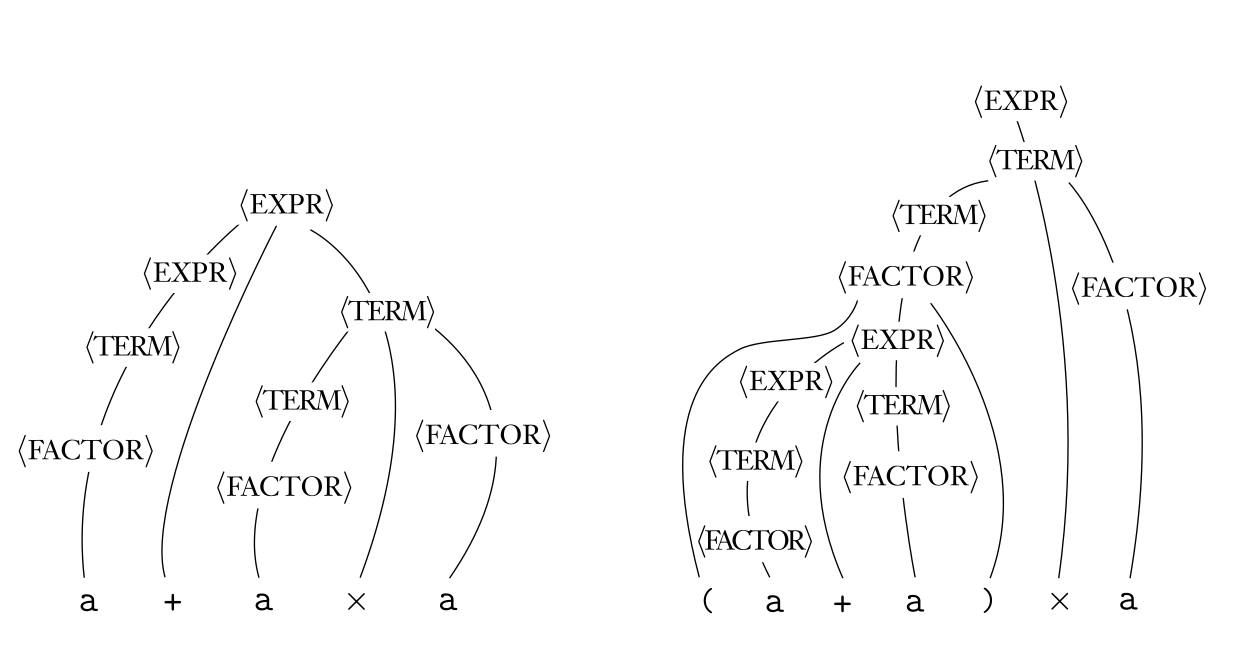leftmost derivation

• Derivation that at every step the leftmost remaining variable is the one replaced

AMBIGUITY

DEF

• A string w is derived ambiguously in context-free grammar G if it has two or more different leftmost derivations.
• Grammar G is ambiguous if it generates some string ambiguously.

CHOMSKY NORMAL FORM

Simplified form for CFG, can generate all CFL.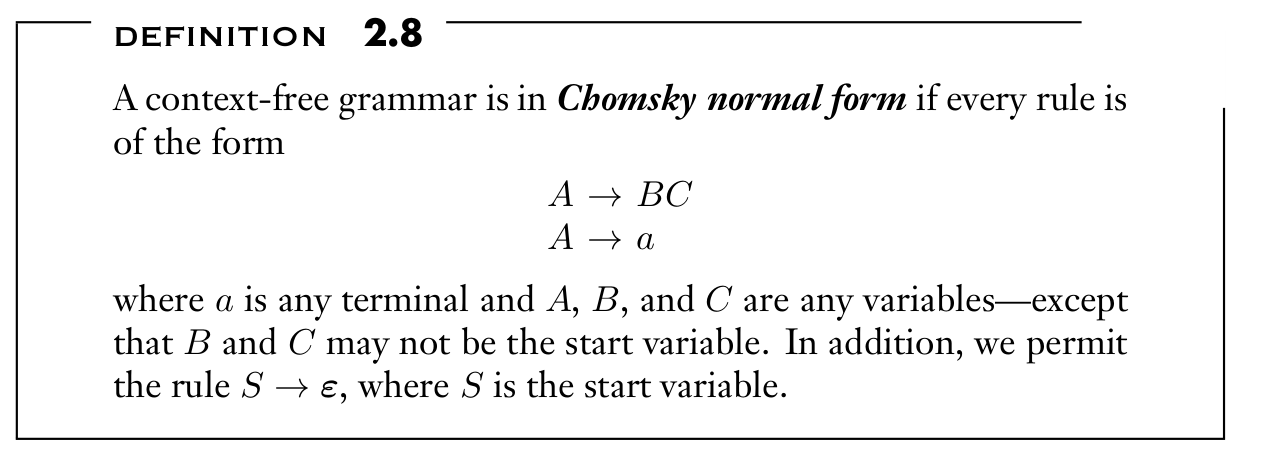2.2 PUSHDOWN AUTOMATA

nondeterministic PDA (with extra stack )

• DEF
• (transition functions return sets)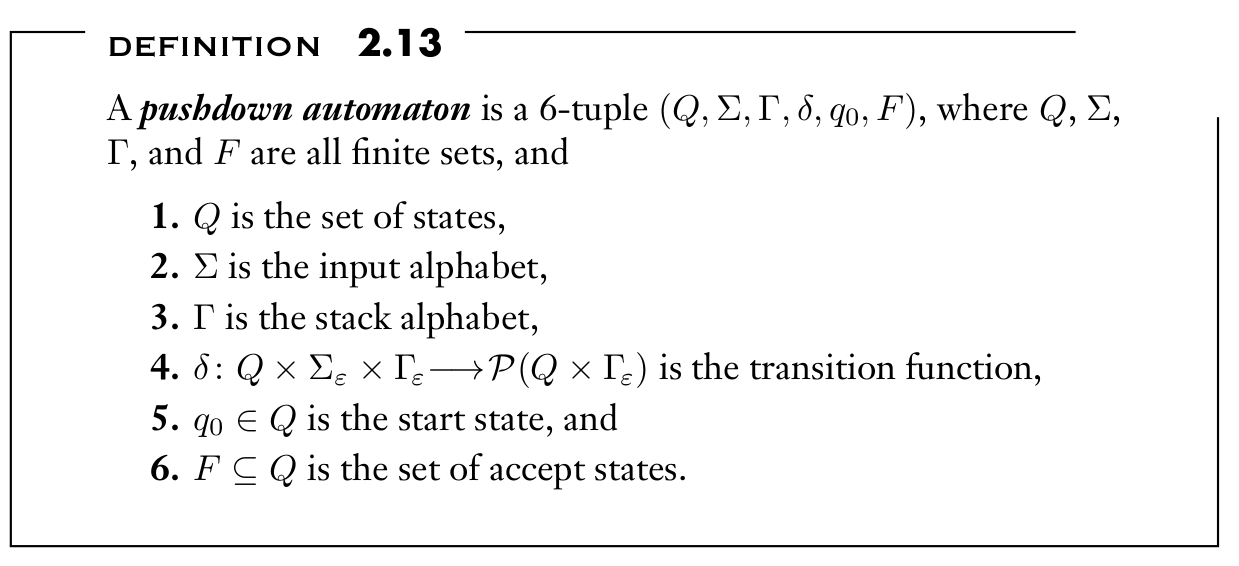Computation under PDA

• A pushdown automaton $M = (Q, Σ, Γ, δ, q_0 , F )$ while input $\in$ $Σ_ε$, states $\in Q$, stack strings $\in Γ^{ ∗ }$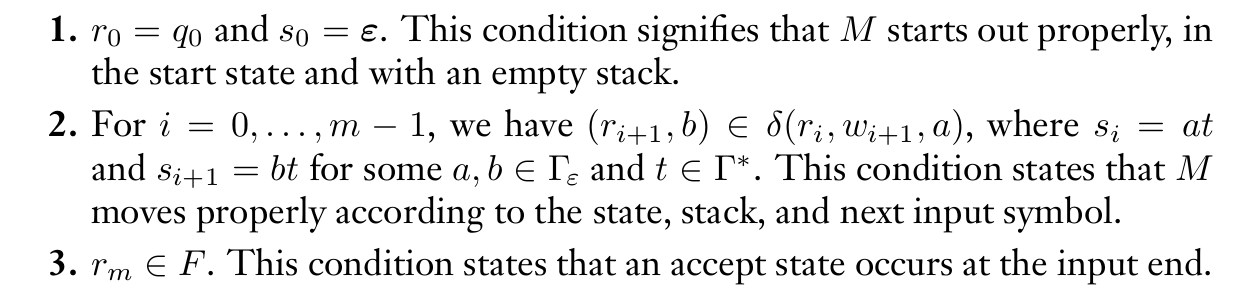Example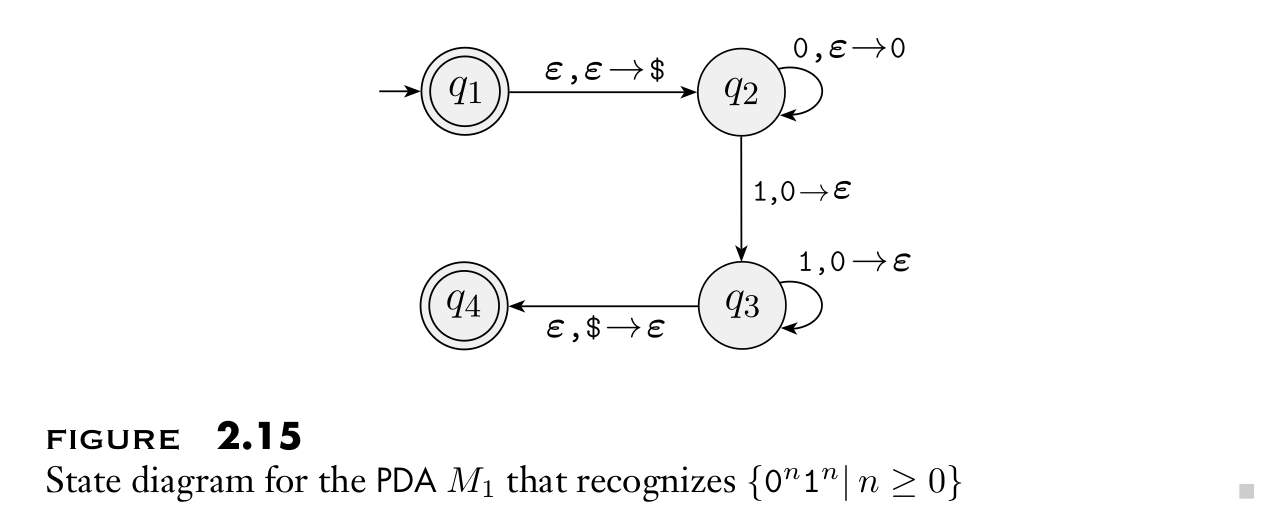EQUIVALENCE WITH CONTEXT-FREE GRAMMARS

• 2.21 If a language is context free, then some pushdown automaton recognizes it.
• 2.27 If a pushdown automaton recognizes some language, then it is context free.

2.21

• Construct PDA P to accept all strings generated by CFG G

Description of P

1. stack push: marker $than Start variable 2. while ( stack.top != ‘\$’ )
• (stack.top == Variable A) nondeterministically select one of the rules A –> w and substitute A by w
• (stack.top == Terminal a) read next symbol and compare it to a